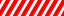# Square Root Calculator Online

Square root calculator online with example. You can find the square root of any number using this tool. It’s very quick, simple and accurate.

 √RelatedThe mathematical world is incomplete without squaring numbers. From childhood to pursuing professional degrees square function is everywhere.

In primary classes, our mathematics teacher instructed us to learn the square root of some numbers. To some extent remembering squares was not a big deal. What’s more, we were taught to manually find the square root. Consequently, our minds became the square root calculator.

Undoubtedly, calculating square roots using the calculator has some advantages over manual calculations. Using the square root calculator you can easily find out the square root of any number in just one click. The Calculator applications on mobile phones may have this feature. However, most of the time it is confusing to use them.

Read the full article to know how to use the tool. Furthermore, you will also get to know the advantages of using it. Therefore, don’t miss any of the sections.

## Mathematics Behind Calculation

The square of any number is multiplying that number by itself. For example, the square of 6 is 36. We straightaway multiplied 6 with 6 to get 36. Now, when we find the square root of 36. It comes out to be 6. Because the square of 6 is 36.

The numbers like 4, 25, 36, etc are perfect squares. Just because their square roots are whole numbers. For example, the square root of 36 is 6 which is a whole number. Similarly, the squares having decimal square roots are not said to be perfect squares.

Somewhat finding the square roots of a perfect square is possible. Consequently, it consumes less time and effort. Whereas on the other hand finding the square root of imperfect squares is difficult.

The square root of any square can be determined by prime factorization (if it’s a perfect square). Firstly, resolve the square number into its prime factors. Then, out of the prime factors will make pairs of all similar factors. Lastly, choose one number from each pair and multiply them. As a result, you get the square root.

Also, it is important to realize that this process only works for finding the square roots for perfect squares. For the other numbers, you can keep the rest prime factor under the root with the answer. Otherwise, solve them in order to find the decimal value. Also, you can find the square root by the LCM trick.

##### Let’s find the square root of 144:

First of all resolve 144 as the product of prime numbers.

In this case it would be 2 x 2 x 2 x 2 x 3 x 3.

Now, as there are six prime numbers hence we will get three pairs: (2 x 2), (2 x 2), and (3 x 3).

Select one number out of all the pairs and multiply them.

In this situation we will get 2 x 2 x 3 = 12.

Obviously, the square of 12 is 144.

##### 1) Square root of 2:

2 = 1.41421356237

You will get 1.41421356237 as an answer.

5 = 2.2360679775

##### 3) Square root of 12:

12 = 3.46410161515

20 = 4.472135955

## Why to use the Square Root Calculator?

There are a number of advantages to choosing the Square Root Calculator over manual calculation.

• Easy and Saves Time: This point doesn’t need to be explained very much. As we know whenever we use the calculator we get answers very fast. Whenever we try solving complex problems ourselves it unquestionably consumes very much time. By using the root calculator you can save time and effort too.
• You can use your calculator any time anywhere whereas on the other hand pen and paper is usually not available at every place. So, in terms of availability square root calculator takes the spot.
• Accuracy: In every calculation, we try to less the number of error as much as possible. When you will perform a calculation by a calculation tool the probability of getting an error result is zero. Why? It is just because the tool uses an algorithm to solve questions. For every input, you give in to the tool the calculator starts processing it and gives the output. If all your inputs are correct you can’t expect the wrong answer.

#### How to use the Square Root Calculator?

The root calculator is an online service. Hence, you would need an Internet connection and a device to access the Internet. After that, just open your web browser and go to Square Root Calculator. Wait until it completely loads up.

Finally, you can see the input box for entering the square. Enter the square for which you want to find out the root. Then simply click on the Calculate button. Your result will appear below. For the new calculation press the Reset button. This was all about the Square Root Calculator.